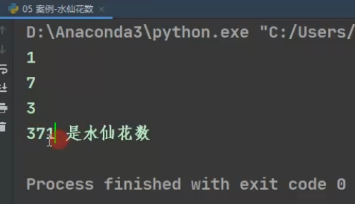## Python入门初学九、数值运算（附水仙花案例）

### 1、算数运算符

``````three = 3
ten = 10

print(ten % three)
``````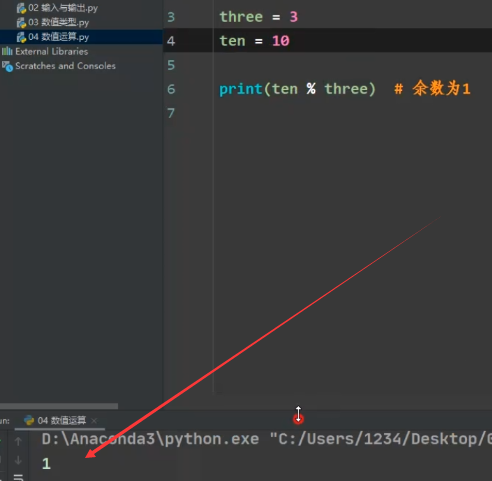``````three = 3
ten = 10

print(ten // three)
``````

10 除以 3 以后，结果为 3.33333 无限循环，然后用两个// 作为运算，表示取整，那么结果就是3 。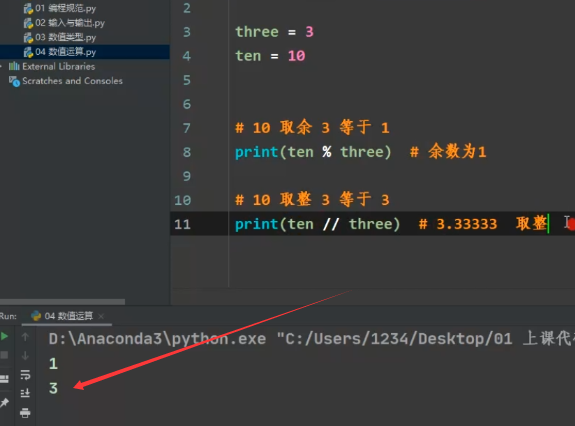``````three = 3
ten = 10

print(ten ** three)
``````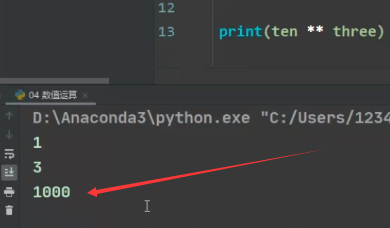+ 10 + 20 = 30
- 10 - 20 = 10
* 10 * 20 = 200
/ 10 / 20 = 0.5
// 取整除 返回除法的整数部分（商）9 // 2 输出结果 4
% 取余数 返回除法的余数 9 % 2 = 1
** 又称次方、乘方，2 ** 3 = 8

• 先乘除后加减
• 同级运算符是 从左到右 计算
• 可以使用 () 调整计算的优先级

** 幂（最高优先级）
*/%// 乘、除、取余数、取整数
+ - 加法、减法

``````2 + 3 * 5 = 17
(2 + 3) * 5 = 25
2 * 3 + 5 = 11
2 * (3 + 5) = 16
``````

### 2、赋值运算符

``````ten += three
``````

ten 和 three 两个变量相加，通过 += 这个赋值运算符号，把结果赋给左边的变量。

``````print ('-------------------------------------------------------------------')
ten += three

print(ten)
``````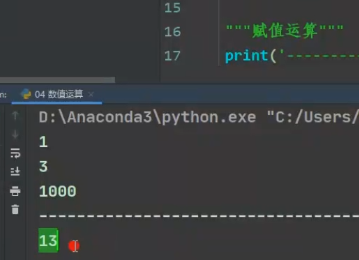= 简单的赋值运算符 c = a + b 将 a + b 的运算结果赋值为 c
+= 加法赋值运算符 c += a 等效于 c = c + a
-= 减法赋值运算符 c -= a 等效于 c = c - a
*= 乘法赋值运算符 c *= a 等效于 c = c * a
/= 除法赋值运算符 c /= a 等效于 c = c / a
%= 取模赋值运算符 c %= a 等效于 c = c % a
**= 幂赋值运算符 c **= a 等效于 c = c ** a
//= 取整赋值运算符 c //= a 等效于 c = c // a

### 3、水仙花案例

###### 1）取个位数

371定义一个number

``````number = 371
``````

``````number = 371

g = number % 10
print(g)
``````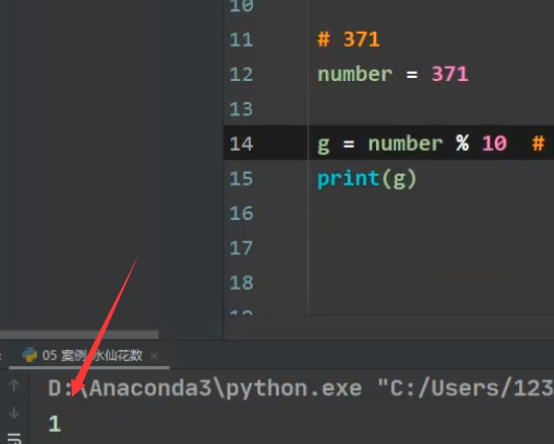###### 2）取十位数

``````number / 10 % 10
``````

``````number = 371

s = number / 10 % 10
print(s)
``````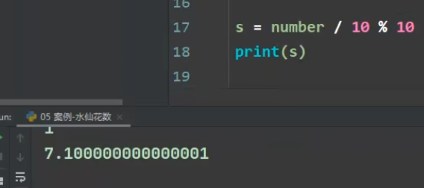``````number = 371

s = int(number / 10 % 10)
print(s)
``````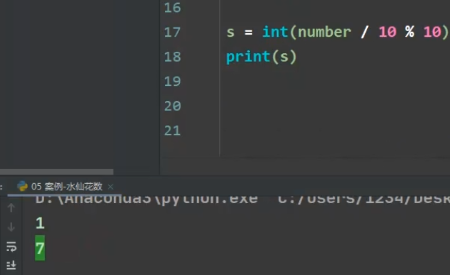###### 3）取百位数

``````number = 371

b = number // 100
print(b)
``````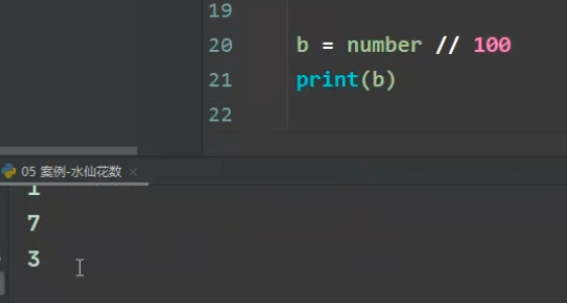###### 4）判断水仙花

``````number = 371

g = number % 10
print(g)

s = int(number / 10 % 10)
print(s)

b = number // 100
print(b)

if number == g ** 3 + s ** 3 + b ** 3:
print(number,'是水仙花数')
``````